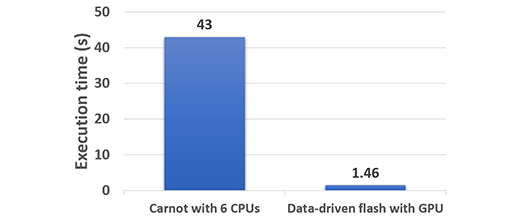A large number of simulators, whether they relate to the design of reaction processes, the evolution of oil reservoirs or combustion systems, require access to thermodynamic properties. In order to provide these properties, IFPEN has been developing a library of calculation modules, called “Carnot”, named after the famous French thermodynamics expert. These calculations, in particular those concerning phase equilibrium (also known as flash calculations), generally require the use of substantial calculation resources due to the complexity of the systems considered, and represent in many cases the most time-consuming step in the simulation process.

To address this, a PhD research project1 set about developing a data-driven learning algorithm, leveraging neural networks, with a view to substituting it for existing flash calculations . Three specific neural networks were established (figure 1) to:

• predict the number and type of coexisting equilibrium phases;

• initialize the distribution coefficients2,K_i;

• evaluate the fugacity coefficients3, φ_i used to update K_i.

Click on the picture to enlarge

The need to scale up equilibrium calculations highlights the benefits of data-driven flash computations since one of the most compelling advantages of neural networks is that they lend themselves to parallel computation.

Our methodology was validated for a panel of experiments which delivered a 30-fold speedup in computation time (figure 2), while maintaining a high degree of accuracy.Figure 2: Comparison between the Carnot tool and data-driven flash in terms of execution time for 230,000 samples of a water/methane mixture.

The next steps will target the automation of the learning framework, for any given composition, and the integration into Carnot of the resulting statistical models, in order to evaluate the performance on simulations based on reference compositions.

In the longer term, the objective will be to optimize the learning process in real-time under the operating conditions specific to each numerical simulation scenario.

1- Jingang QU: “Acceleration of numerical simulations by means of deep learning - Application to thermodynamic equilibrium calculations”, ongoing IFPEN thesis
2- The distribution coefficient of the i-th component K_i corresponds to the ratio of the molar fraction of the i-th component between the gas and liquid phase.
3- The fugacity coefficient of the i-th component φ_i is the ratio between the real fugacity and the fugacity of the perfect gas in a mixture with the same pressure, temperature and composition.

Publication:

  J. Qu, M. D’Heilly, P. Gallinari, J-C. de Hemptinne, T. Faney et S. Youssef, Efficient phase equilibrium computations using learning algorithms, ESAT 2021-31st European Symposium on Applied Thermodynamics, July 2021.

Scientific contact: thibault.faney@ifpen.fr